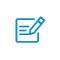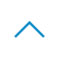•• 注册 忘记密码?

# 机器学习中需要了解的 5 种基础采样方法

WalmartLabs 的数据科学家 Rahul Agarwal 提出了数据科学家需要了解的 5 种基础采样方法，包括简单随机抽样、分层采样、水塘采样、随机欠采样和过采样等。采样是数据科学中常见问题，工业机器人数据采样中也会用到。

sample_df = df.sample(100)A 镇有 100 万工人，

B 镇有 200 万工人，以及

C 镇有 300 万退休人员。

from sklearn.model_selection import train_test_splitX_train, X_test, y_train, y_test = train_test_split(X, y, stratify=y,  test_size=0.25)import randomdef generator(max):

number = 1

while number < max:

number += 1

yield number# Create as stream generator

stream = generator(10000)# Doing Reservoir Sampling from the stream

k=5

reservoir = []

for i, element in enumerate(stream):

if i+1<= k:

reservoir.append(element)

else:

probability = k/(i+1)

if random.random() < probability:

# Select item in stream and remove one of the k items already selected

reservoir[random.choice(range(0,k))] = elementprint(reservoir)

------------------------------------

[1369, 4108, 9986, 828, 5589]

2/3*1/2 = 1/3

1–1/3=2/3from sklearn.datasets import make_classificationX, y = make_classification( n_classes=2, class_sep=1.5, weights=[0.9, 0.1], n_informative=3, n_redundant=1, flip_y=0, n_features=20, n_clusters_per_class=1, n_samples=100, random_state=10)X = pd.DataFrame(X)X[ target ] = y

num_0 = len(X[X[ target ]==0])num_1 = len(X[X[ target ]==1])print(num_0,num_1)# random undersampleundersampled_data = pd.concat([ X[X[ target ]==0].sample(num_1) , X[X[ target ]==1] ])print(len(undersampled_data))# random oversampleoversampled_data = pd.concat([ X[X[ target ]==0] , X[X[ target ]==1].sample(num_0, replace=True) ])print(len(oversampled_data))------------------------------------------------------------OUTPUT:90 1020180

imbalanced-learn（imblearn）是一个用于解决不平衡数据集问题的 python 包，它提供了多种方法来进行欠采样和过采样。tl = TomekLinks(return_indices=True, ratio= majority )

X_tl, y_tl, id_tl = tl.fit_sample(X, y)

b. 使用 SMOTE 进行过采样：

from imblearn.over_sampling import SMOTE

smote = SMOTE(ratio= minority )

X_sm, y_sm = smote.fit_sample(X, y)

imbLearn 包中还有许多其他方法，可以用于欠采样（Cluster Centroids, NearMiss 等）和过采样（ADASYN 和 bSMOTE）。扫码入群返回顶部